# Volume + time - math problems

#### Number of problems found: 59

• Volume per timeHow long does fill take for a pump with a volume flow of 200 l per minute fill a cube-shaped tank up to 75% of its height if the length of the cube edge is 4 m?
• Volume of woodEvery year, at the same time, an increase in the volume of wood in the forest is measured. The increase is regularly p% compared to the previous year. If in 10 years the volume of wood has increased by 10%, what is the number p?
• RC time constant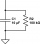You introduced 1 Coulomb worth of electrons into the inner volume of a dielectric material with ϵr=6. 30 minutes later, you found that only 36.79% of the electrons were in the inner volume. Determine the conductivity σ of the dielectric material.
• 40% volume40% volume with 104 uph (units per labor hour) 8 people working. What is the volume?
• The dam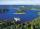The water reservoir is filled with first tributary for 25 hours second tributary for 35 hours. Some time were both inlets open, then a second tributary closed and the tank was filled in four hours. What time water flowed from both tributaries? (Expressed
• A filterIt is a pool with a volume of 3500 liters. The filter filters at 4m cubic per hour. How many minutes would it filter the entire pool?
• Pump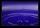From the pump in the yard dripping water. Every drop is 1 ml. How many drops will be necessary before filling a bucket with a volume of 10 l? Calculate how long it will fulfill the bucket when drops fall each second.
• Tank 9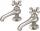The tank with volume V is filled with one pump for three hours and by second pump for 5 hours. When both pumps will run simultaneously calculate: a) how much of the total volume of the tank is filled in one hour b) for how long is the tank full
• The wellbore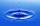The wellbore has a tributary of 2 m3 per hour. When there is no tapping, there are a stable 28 liters of water in the well. The pump suction basket is at the bottom of the well. At 14.00, the water was pumped out at a rate of 0.5 liters of water every se
• Pumps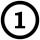First pump flows 16 liters per second into the basin by the second pump 75% of the first and by third pump half more than the second. How long will take fill basin by all three pumps simultaneously volume 15 m3 (cubic meters)?
• Water pool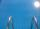Pool with volume 990hl completely filled, if water flows by one tap 8 hours and by second tap 6 hours. First tap give 10hl more than second per hour. How many hl flows in each of them in an hour?
• Three pumpsWe are filling the pool. The first pump would be filled in 12 hours, the second pump in 15 hours. If all three pumps were running at the same time, it would fill the pool for 4 hours. How long would the pool fill only with the third pump?
• Inlet and outletThe pool has a capacity of 50 hl. The inlet pipe flows in 1 minute 1.25 hl, and the waste pipe outlet is draining the full pool in 50 minutes. How long will take the empty the full pool when both the inlet and outlet are opened at the same time?
• Two pipesHow long will the pool be filled with a double supply pipe if it takes the pool to fill the first pipe by 4 hours longer and the second pipe 9 hours longer than both pipes open at the same time?
• Blueberries collecting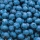Marie collects blueberries 2 l in 1 hour. Began collecting at 8.00 pm, Joe collects 1.5 l in 1 hour. Peter 1 l for 1 hr. And they began to gather at 08.30 pm. Together they collect 10 l. At what time will they all have collected?
• Two water containers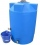In the first container, there are 200 m3 of water and in the second 40 m3. The first container will flow down at a rate of 10 m3 water per hour. At the same time flows to the second rate of 5 m3 per hour. After how many hours there will be three times les
• The cylindrical container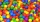The cylindrical container has a base area of 300 cm3 and a height of 10 cm. It is 90% filled with water. We gradually insert metal balls into the water, each with a volume of 20 cm3. After inserting how many balls for the first time does water flow over
• Workers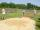Workers digging a jump pit in the school yard. Pit has a cuboid shape with a length 12 m, a width 20 dm and depth 36 cm. They excavate 0.4 cubic meters of soil an hour. How much time (hours and minutes) is need to the excavate this pit?
• Tank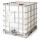How many minutes does it take to fill the tank to 25 cubic meters of water filled 150hl per hour?
• Pump680 liters of water were pumped in 8 minutes. How many liters was spent in 56 minutes?

Do you have an interesting mathematical word problem that you can't solve it? Submit a math problem, and we can try to solve it.

We will send a solution to your e-mail address. Solved examples are also published here. Please enter the e-mail correctly and check whether you don't have a full mailbox.

Please do not submit problems from current active competitions such as Mathematical Olympiad, correspondence seminars etc...

Tip: Our volume units converter will help you with the conversion of volume units. Do you want to convert time units like minutes to seconds? Volume - math word problems. Time - math word problems.